Mark Dawes

•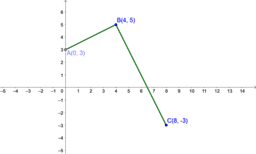Prove right angle

Activity

Mark Dawes

•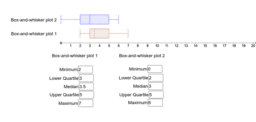Box-and-whisker plot 2

Activity

Mark Dawes

•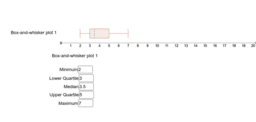Box-and-whisker plot 1

Activity

Mark Dawes

•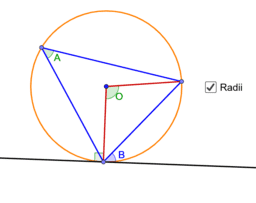Circle Theorem 6

Activity

Mark Dawes

•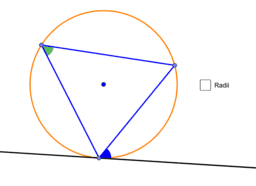Circle Theorem 6

Activity

Mark Dawes

•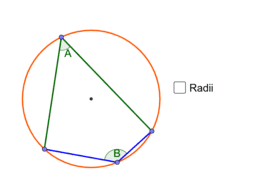Circle Theorems 5

Activity

Mark Dawes

•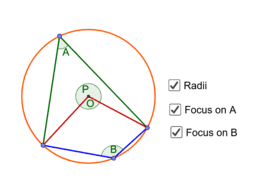Circle Theorems 5

Activity

Mark Dawes

•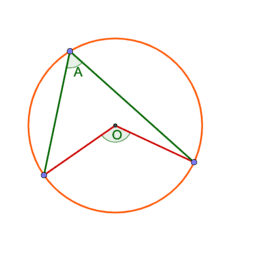Circle Theorems 5

Activity

Mark Dawes

•Circle Theorems 4

Activity

Mark Dawes

•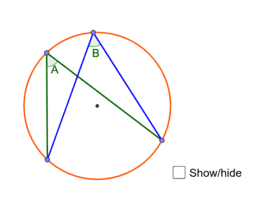Circle Theorems 3

Activity

Mark Dawes

•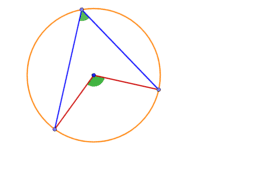Circle Theorem 2

Activity

Mark Dawes

•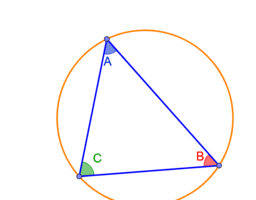Circle Theorem 1

Activity

Mark Dawes

•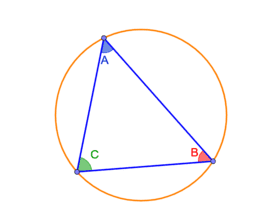Circle Theorem 1

Activity

Mark Dawes

•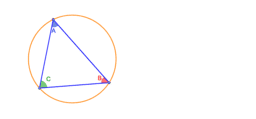Circle Theorem 1

Activity

Mark Dawes

•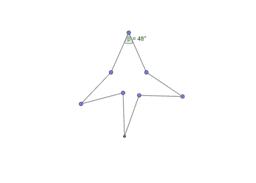48deg

Activity

Mark Dawes

•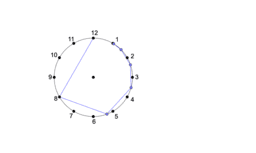Clockface - divide

Activity

Mark Dawes

•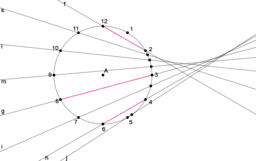Clockface mult to 24 - v2

Activity

Mark Dawes

•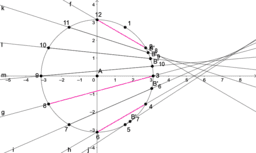Clockface prod-24

Activity

Mark Dawes

•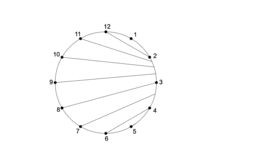Clockface mult to 24

Activity

Mark Dawes

•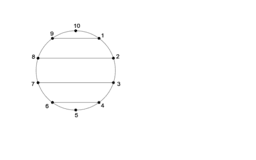Clockface-10 blank

Activity

Mark Dawes

•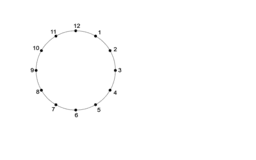Clockface blank

Activity

Mark Dawes

•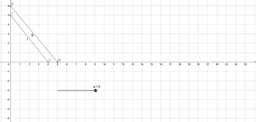Parallel q-mark

Activity

Mark Dawes

•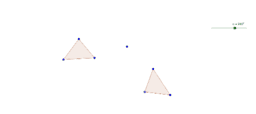Rotation

Activity

Mark Dawes

•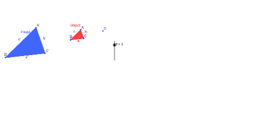Activity

Mark Dawes